Скачать презентацию Transport Fundamentals n n n This chapter considers

9907284f9d6b722a6d69ef04fabf4c8e.ppt

• Количество слайдов: 21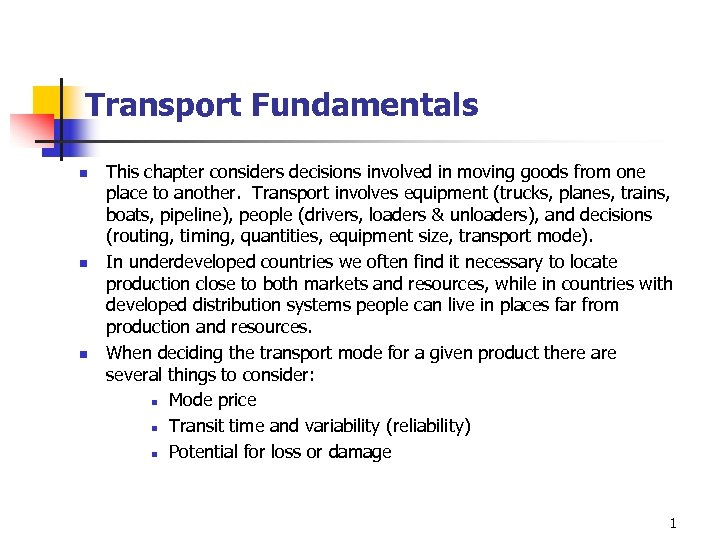Transport Fundamentals n n n This chapter considers decisions involved in moving goods from one place to another. Transport involves equipment (trucks, planes, trains, boats, pipeline), people (drivers, loaders & unloaders), and decisions (routing, timing, quantities, equipment size, transport mode). In underdeveloped countries we often find it necessary to locate production close to both markets and resources, while in countries with developed distribution systems people can live in places far from production and resources. When deciding the transport mode for a given product there are several things to consider: n Mode price n Transit time and variability (reliability) n Potential for loss or damage 1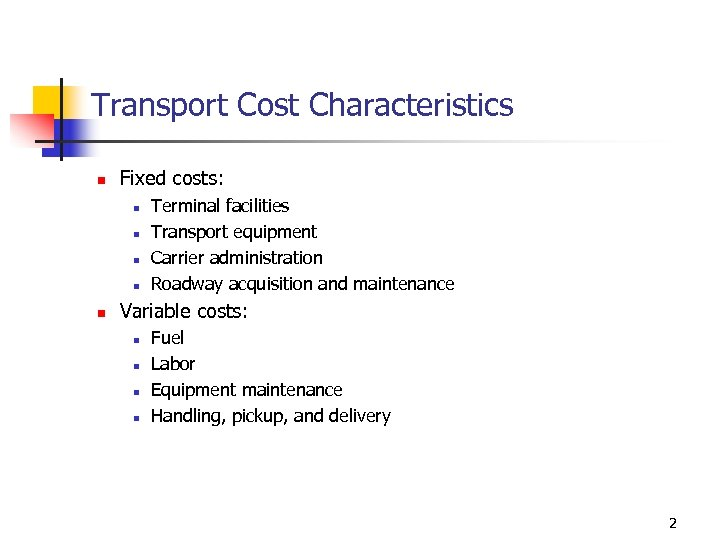Transport Cost Characteristics n Fixed costs: n n n Terminal facilities Transport equipment Carrier administration Roadway acquisition and maintenance Variable costs: n n Fuel Labor Equipment maintenance Handling, pickup, and delivery 2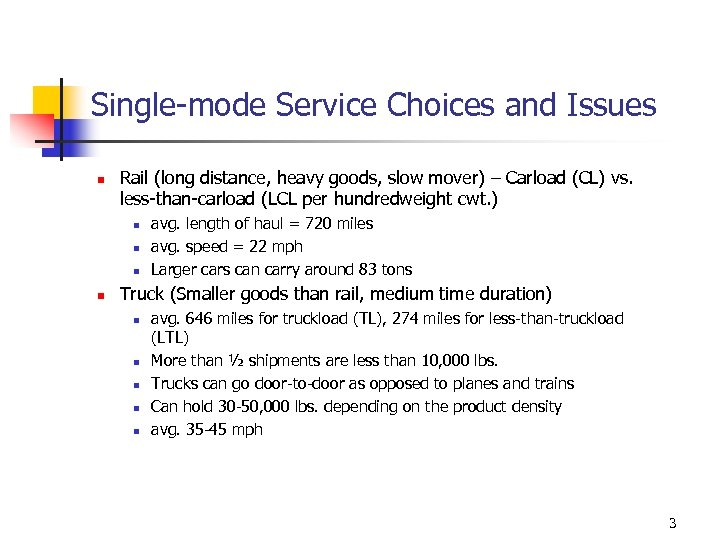Single-mode Service Choices and Issues n Rail (long distance, heavy goods, slow mover) – Carload (CL) vs. less-than-carload (LCL per hundredweight cwt. ) n n avg. length of haul = 720 miles avg. speed = 22 mph Larger cars can carry around 83 tons Truck (Smaller goods than rail, medium time duration) n n n avg. 646 miles for truckload (TL), 274 miles for less-than-truckload (LTL) More than ½ shipments are less than 10, 000 lbs. Trucks can go door-to-door as opposed to planes and trains Can hold 30 -50, 000 lbs. depending on the product density avg. 35 -45 mph 3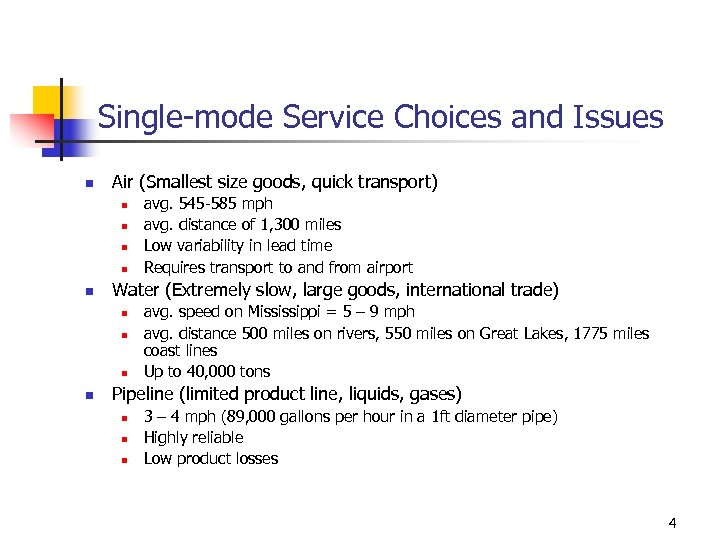Single-mode Service Choices and Issues n Air (Smallest size goods, quick transport) n n n Water (Extremely slow, large goods, international trade) n n avg. 545 -585 mph avg. distance of 1, 300 miles Low variability in lead time Requires transport to and from airport avg. speed on Mississippi = 5 – 9 mph avg. distance 500 miles on rivers, 550 miles on Great Lakes, 1775 miles coast lines Up to 40, 000 tons Pipeline (limited product line, liquids, gases) n n n 3 – 4 mph (89, 000 gallons per hour in a 1 ft diameter pipe) Highly reliable Low product losses 4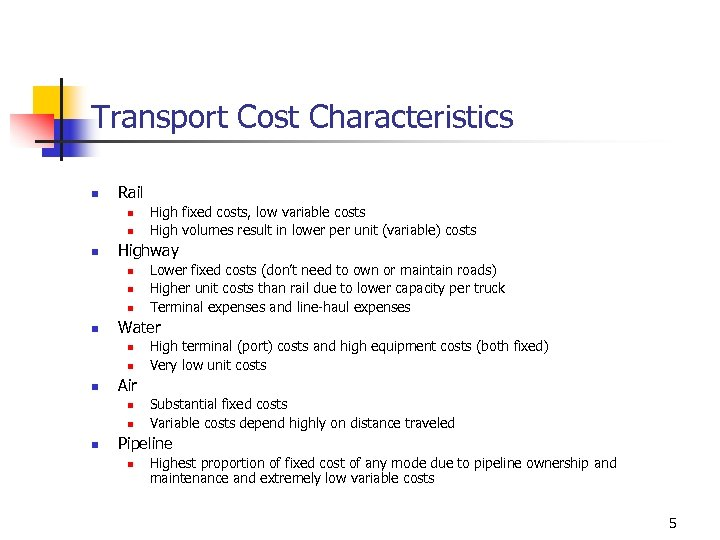Transport Cost Characteristics n Rail n n n Highway n n n High terminal (port) costs and high equipment costs (both fixed) Very low unit costs Air n n n Lower fixed costs (don’t need to own or maintain roads) Higher unit costs than rail due to lower capacity per truck Terminal expenses and line-haul expenses Water n n High fixed costs, low variable costs High volumes result in lower per unit (variable) costs Substantial fixed costs Variable costs depend highly on distance traveled Pipeline n Highest proportion of fixed cost of any mode due to pipeline ownership and maintenance and extremely low variable costs 5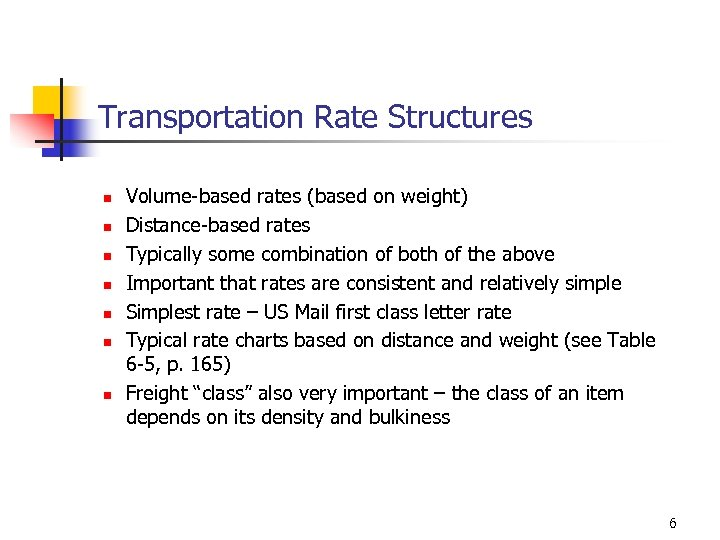Transportation Rate Structures n n n n Volume-based rates (based on weight) Distance-based rates Typically some combination of both of the above Important that rates are consistent and relatively simple Simplest rate – US Mail first class letter rate Typical rate charts based on distance and weight (see Table 6 -5, p. 165) Freight “class” also very important – the class of an item depends on its density and bulkiness 6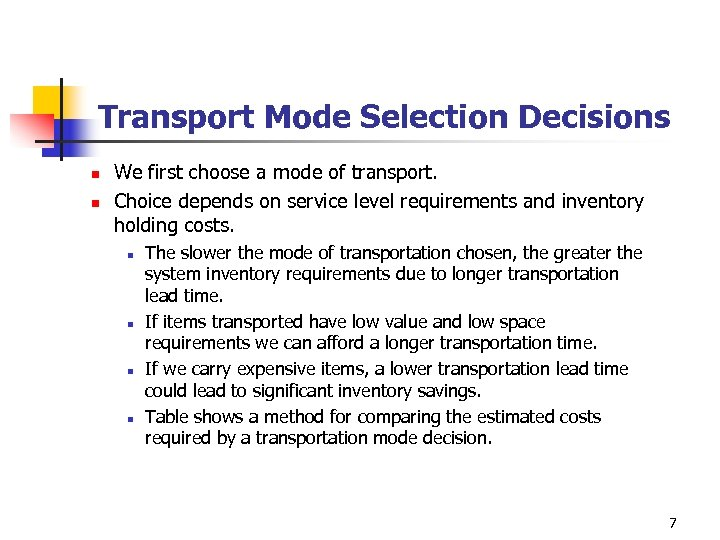Transport Mode Selection Decisions n n We first choose a mode of transport. Choice depends on service level requirements and inventory holding costs. n n The slower the mode of transportation chosen, the greater the system inventory requirements due to longer transportation lead time. If items transported have low value and low space requirements we can afford a longer transportation time. If we carry expensive items, a lower transportation lead time could lead to significant inventory savings. Table shows a method for comparing the estimated costs required by a transportation mode decision. 7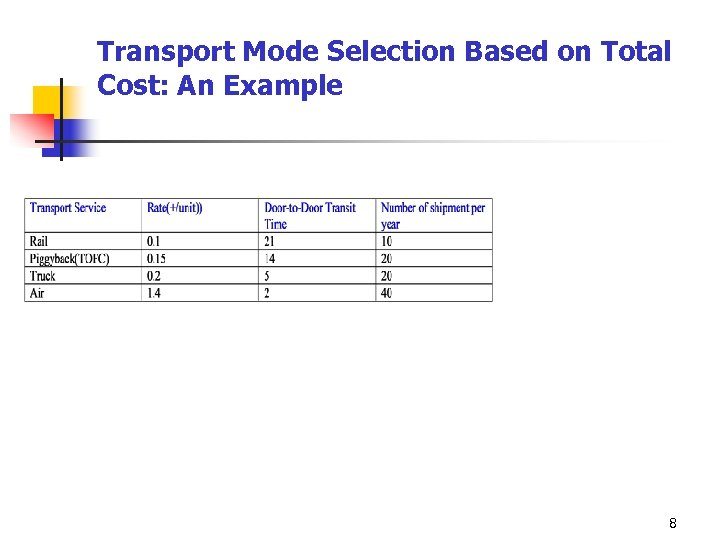Transport Mode Selection Based on Total Cost: An Example 8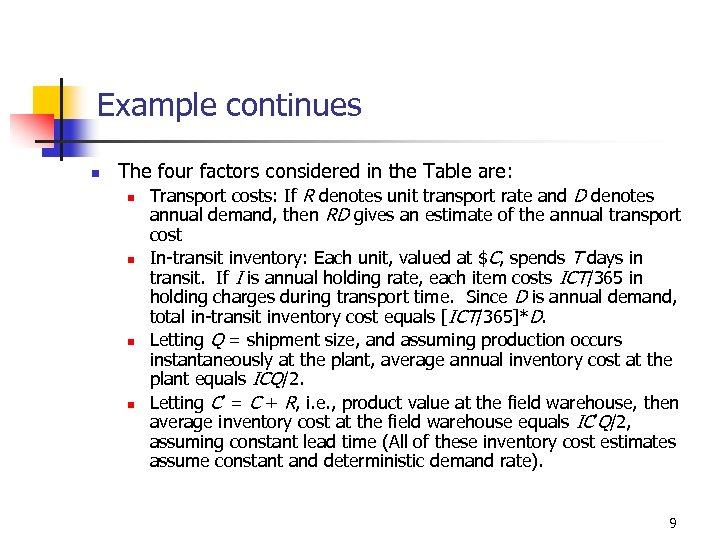Example continues n The four factors considered in the Table are: n Transport costs: If R denotes unit transport rate and D denotes annual demand, then RD gives an estimate of the annual transport n n n cost In-transit inventory: Each unit, valued at \$C, spends T days in transit. If I is annual holding rate, each item costs ICT/365 in holding charges during transport time. Since D is annual demand, total in-transit inventory cost equals [ICT/365]*D. Letting Q = shipment size, and assuming production occurs instantaneously at the plant, average annual inventory cost at the plant equals ICQ/2. Letting C’ = C + R, i. e. , product value at the field warehouse, then average inventory cost at the field warehouse equals IC’Q/2, assuming constant lead time (All of these inventory cost estimates assume constant and deterministic demand rate). 9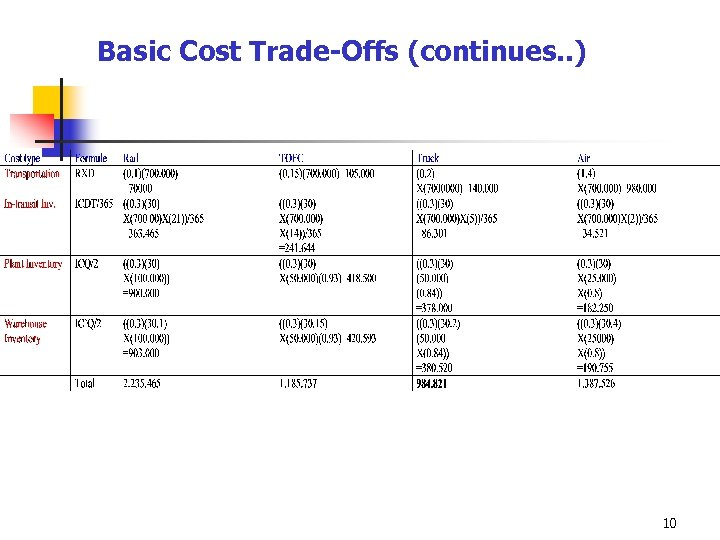Basic Cost Trade-Offs (continues. . ) 10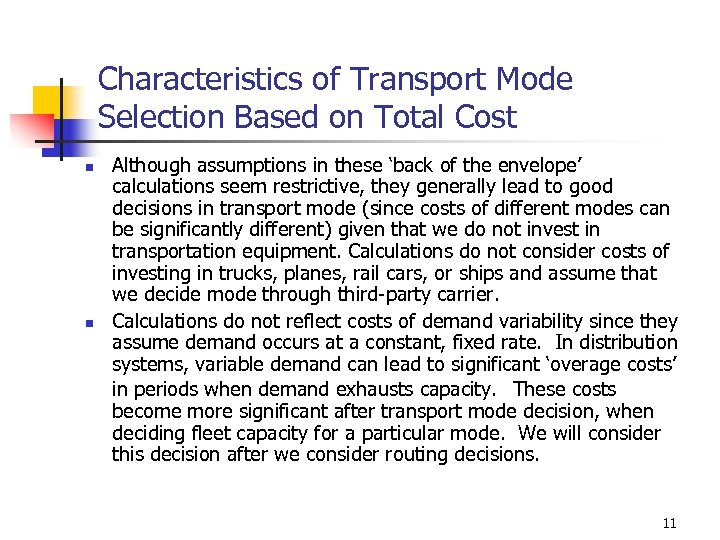Characteristics of Transport Mode Selection Based on Total Cost n n Although assumptions in these ‘back of the envelope’ calculations seem restrictive, they generally lead to good decisions in transport mode (since costs of different modes can be significantly different) given that we do not invest in transportation equipment. Calculations do not consider costs of investing in trucks, planes, rail cars, or ships and assume that we decide mode through third-party carrier. Calculations do not reflect costs of demand variability since they assume demand occurs at a constant, fixed rate. In distribution systems, variable demand can lead to significant ‘overage costs’ in periods when demand exhausts capacity. These costs become more significant after transport mode decision, when deciding fleet capacity for a particular mode. We will consider this decision after we consider routing decisions. 11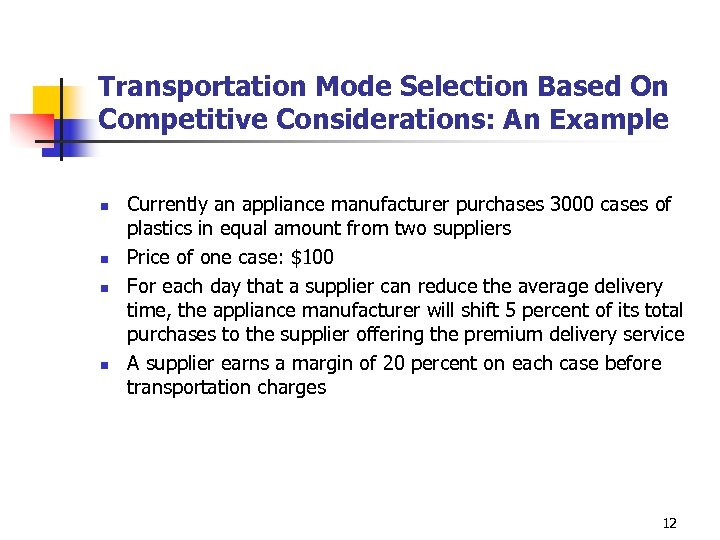Transportation Mode Selection Based On Competitive Considerations: An Example n n Currently an appliance manufacturer purchases 3000 cases of plastics in equal amount from two suppliers Price of one case: \$100 For each day that a supplier can reduce the average delivery time, the appliance manufacturer will shift 5 percent of its total purchases to the supplier offering the premium delivery service A supplier earns a margin of 20 percent on each case before transportation charges 12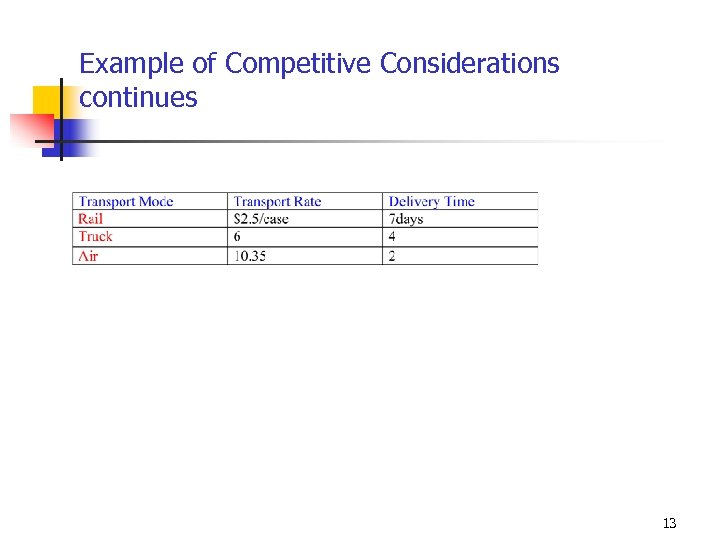Example of Competitive Considerations continues 13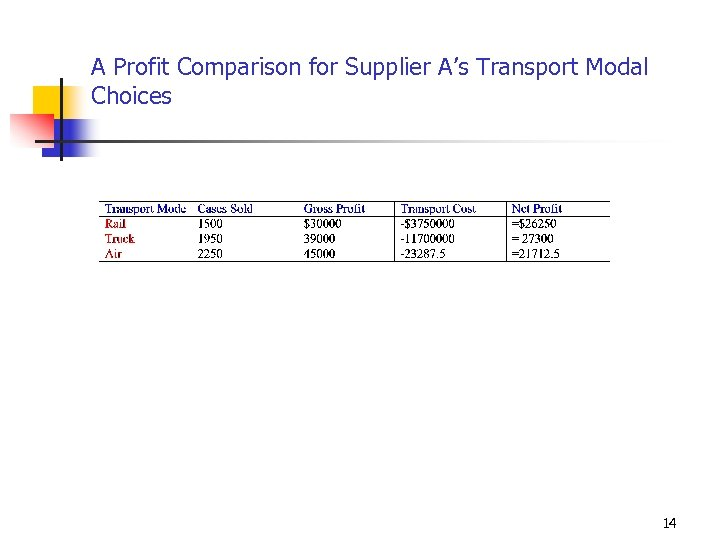A Profit Comparison for Supplier A’s Transport Modal Choices 14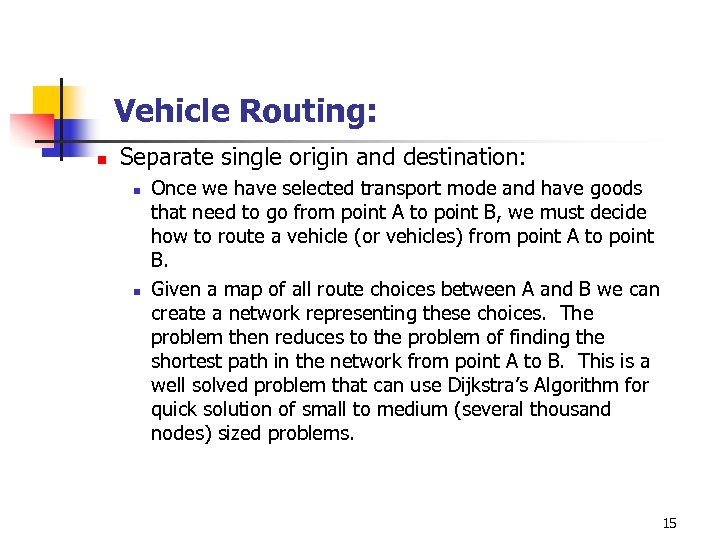Vehicle Routing: n Separate single origin and destination: n n Once we have selected transport mode and have goods that need to go from point A to point B, we must decide how to route a vehicle (or vehicles) from point A to point B. Given a map of all route choices between A and B we can create a network representing these choices. The problem then reduces to the problem of finding the shortest path in the network from point A to B. This is a well solved problem that can use Dijkstra’s Algorithm for quick solution of small to medium (several thousand nodes) sized problems. 15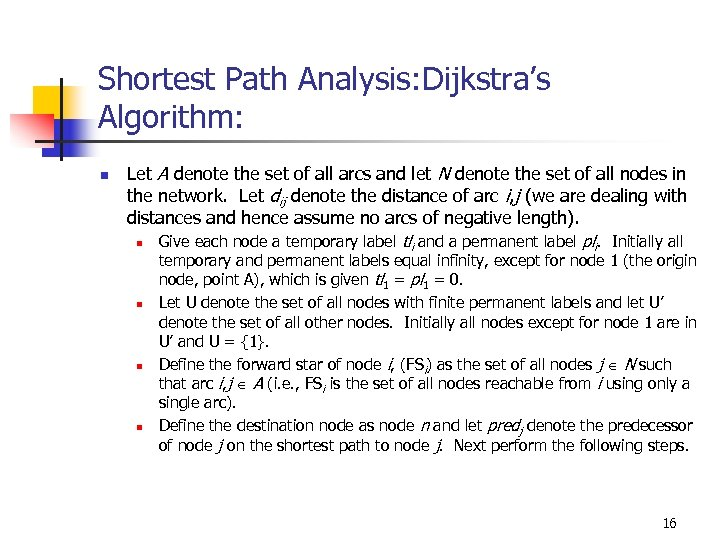Shortest Path Analysis: Dijkstra’s Algorithm: n Let A denote the set of all arcs and let N denote the set of all nodes in the network. Let dij denote the distance of arc i, j (we are dealing with distances and hence assume no arcs of negative length). n n Give each node a temporary label tli and a permanent label pli. Initially all temporary and permanent labels equal infinity, except for node 1 (the origin node, point A), which is given tl 1 = pl 1 = 0. Let U denote the set of all nodes with finite permanent labels and let U’ denote the set of all other nodes. Initially all nodes except for node 1 are in U’ and U = {1}. Define the forward star of node i, (FSi) as the set of all nodes j N such that arc i, j A (i. e. , FSi is the set of all nodes reachable from i using only a single arc). Define the destination node as node n and let predj denote the predecessor of node j on the shortest path to node j. Next perform the following steps. 16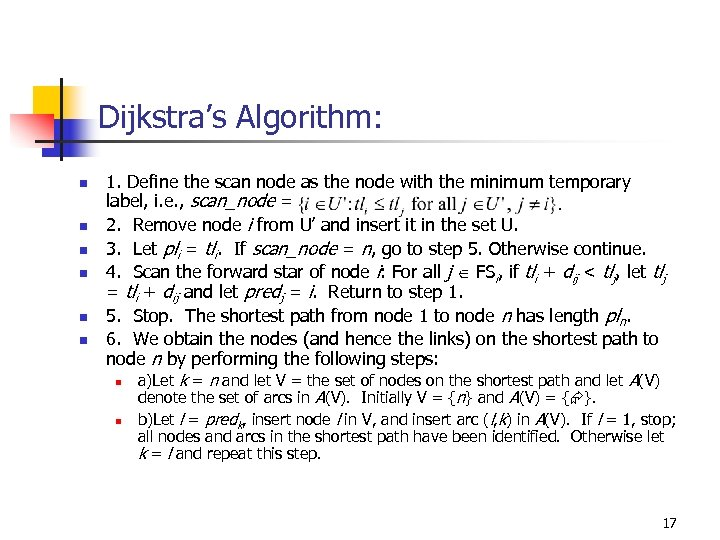Dijkstra’s Algorithm: n n n 1. Define the scan node as the node with the minimum temporary label, i. e. , scan_node = 2. Remove node i from U’ and insert it in the set U. 3. Let pli = tli. If scan_node = n, go to step 5. Otherwise continue. 4. Scan the forward star of node i: For all j FSi, if tli + dij < tlj, let tlj = tli + dij and let predj = i. Return to step 1. 5. Stop. The shortest path from node 1 to node n has length pln. 6. We obtain the nodes (and hence the links) on the shortest path to node n by performing the following steps: n n a)Let k = n and let V = the set of nodes on the shortest path and let A(V) denote the set of arcs in A(V). Initially V = {n} and A(V) = { }. b)Let l = predk, insert node l in V, and insert arc (l, k) in A(V). If l = 1, stop; all nodes and arcs in the shortest path have been identified. Otherwise let k = l and repeat this step. 17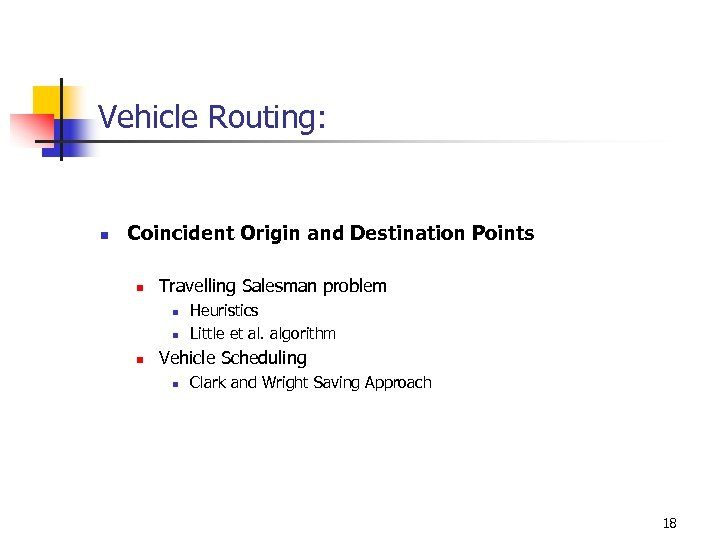Vehicle Routing: n Coincident Origin and Destination Points n Travelling Salesman problem n n n Heuristics Little et al. algorithm Vehicle Scheduling n Clark and Wright Saving Approach 18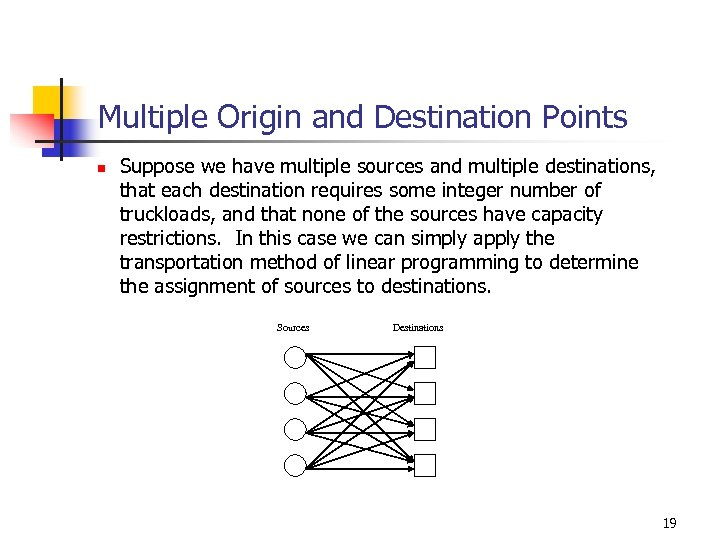Multiple Origin and Destination Points n Suppose we have multiple sources and multiple destinations, that each destination requires some integer number of truckloads, and that none of the sources have capacity restrictions. In this case we can simply apply the transportation method of linear programming to determine the assignment of sources to destinations. Sources Destinations 19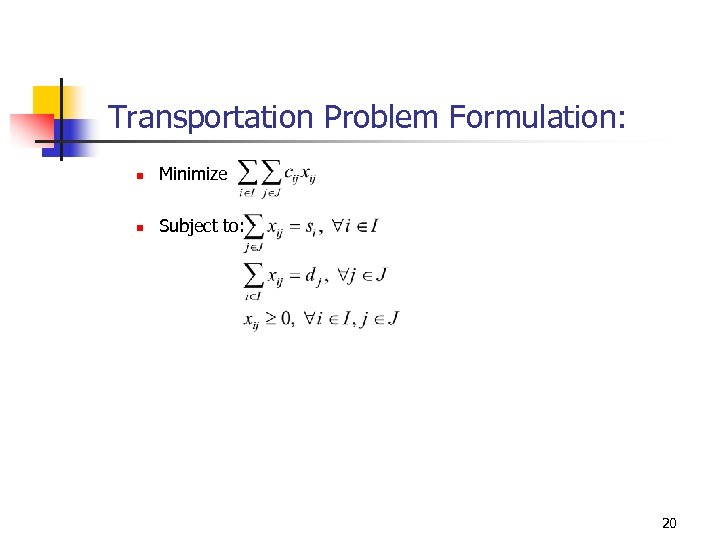Transportation Problem Formulation: n Minimize n Subject to: 20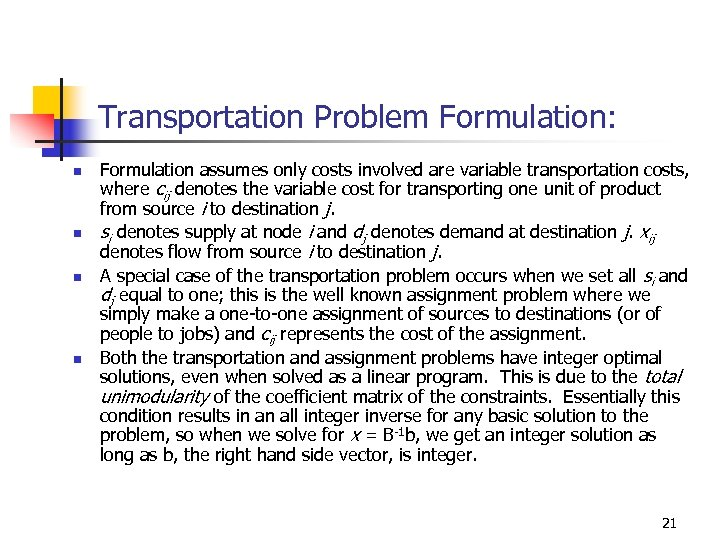Transportation Problem Formulation: n n Formulation assumes only costs involved are variable transportation costs, where cij denotes the variable cost for transporting one unit of product from source i to destination j. si denotes supply at node i and dj denotes demand at destination j. xij denotes flow from source i to destination j. A special case of the transportation problem occurs when we set all si and dj equal to one; this is the well known assignment problem where we simply make a one-to-one assignment of sources to destinations (or of people to jobs) and cij represents the cost of the assignment. Both the transportation and assignment problems have integer optimal solutions, even when solved as a linear program. This is due to the total unimodularity of the coefficient matrix of the constraints. Essentially this condition results in an all integer inverse for any basic solution to the problem, so when we solve for x = B-1 b, we get an integer solution as long as b, the right hand side vector, is integer. 21